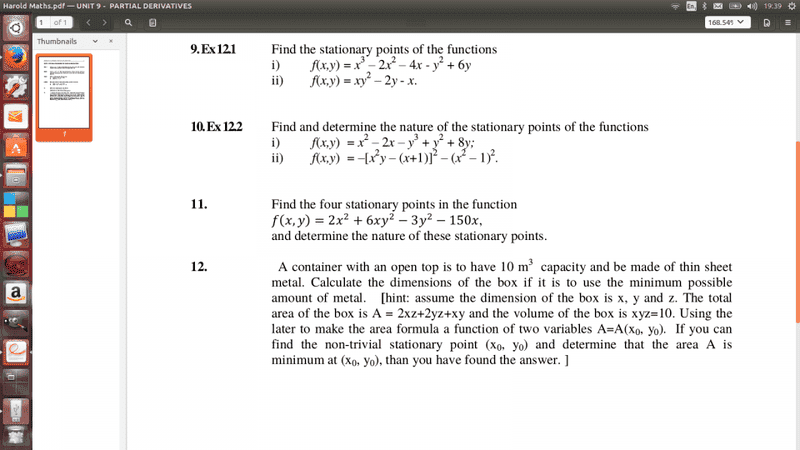# Partial derivative stationary point

## Homework Statement

Hi guys, I am having real trouble with the function 10ii) I can take the derivatives, but I feel like I am missing something, with what I have done. I set $f_x=0$and $f_y=0$ but really cant seem to find away to solve, i keep getting (0,0) which when I plug into wolfram it come out with $$x=0,y=-1/2$$, $$x=-1,y=0$$ and $$x=1, y=-2$$
So this make me think have I done the partial right?## The Attempt at a Solution

[/B]
$$\frac{\partial}{\partial x}=-2(x^2y-x-1)(2xy-1)-2(x^2-1)(2x)$$
$$\frac{\partial}{\partial y}=-2(x^2y-x-1)(x^2)$$

$$-2(x^2y-x-1)(2xy-1)-2(x^2-1)(2x)=0$$
$$-2(x^2y-x-1)(x^2)=0$$

but where do I go from here?

Could someone please give advice, thanks in advance.

## Answers and Replies

BvU
Science Advisor
Homework Helper
I set ##f_x=0\ ## and ##f_y=0##

Two hashes (#) to get inline ##\TeX##, two dollars (\$) to get displayed mathCheck ##f_x## and ##f_y## (*); once OK, ##f_y## gives you something to substitute in ##f_x=0##

(*)  right, thanks Dick(I copied the 2 inside the [ ])

Last edited:
Dick
Science Advisor
Homework Helper
The partials look ok. Think about the y derivative first. It's zero if i) x=0 OR y=?. Fill in the ? with an expression in x. Substitute that into the x derivative.

epenguin
Homework Helper
Gold Member
Not every function of x and y has a stationary point - it is always possible that your function has none.
In that case you will not be able to find it! But then you have to prove it doesn't exist.
In this case I think there is a stationary point.
fy is nicely factorised, which is help. One of the factors is closely related to a bracket in fx which is another help.

Not every condition for one of your derivatives to be 0 necessarily allows the the other to be 0.(Though even if it doesn't, your zeros of one of them still gives information about what the surface f looks like)